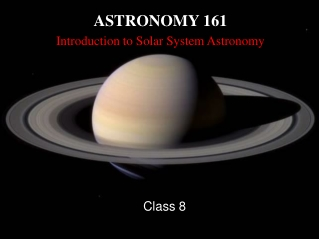DownloadDownload PresentationASTRONOMY 161 Introduction to Solar System Astronomy

# ASTRONOMY 161 Introduction to Solar System Astronomy

Download Presentation## ASTRONOMY 161 Introduction to Solar System Astronomy

- - - - - - - - - - - - - - - - - - - - - - - - - - - E N D - - - - - - - - - - - - - - - - - - - - - - - - - - -
##### Presentation Transcript

1. Applying Newton’s LawsFriday, January 26

2. Applying Newton’s Laws: Key Concepts (1) Newton modified and expanded Kepler’s Laws of Planetary Motion. (2) Kepler described how planets move; Newton explained why they move. (3) Tides are caused by the difference between the Moon’s gravitational force on different sides of the Earth. (4) Tidal forces are slowing the Earth’s rotation & enlarging the Moon’s orbit.

3. (1) Newton modified and expanded Kepler’s Laws of Planetary Motion Kepler’s First Law: The orbits of the planets around the Sun are ellipses with the Sun at one focus. Newton’s revision: The orbits of any pair of objects are conic sections with the center of mass at one focus.

4. As the Earth pulls on Moon, Moon pulls on Earth. Both Earth and Moon orbit the center of mass of the Earth-Moon system: Center of mass = balance point: closer to more massive object.

5. Artificial satellites as envisaged by Isaac Newton: To put an object into orbit, launch it sideways with a large enough speed. How large is large enough?

6. The shape of the orbit depends on the speed of the satellite at launch: Low speed = closed orbit, a circle or ellipse. High speed = open orbit, a parabola or hyperbola. Circles, ellipses, parabolas, and hyperbolas are called conic sections.

7. To remain in a circular orbit just above the Earth’s surface, a satellite must have v = 7.9 km/sec. To attain an open orbit, a satellite must reach at least 11.2 km/sec.

8. Some extra math:

9. Kepler’s Second Law: A line from a planet to the Sun sweeps out equal areas in equal time intervals. Newton’s revision: Angular momentum is conserved.

10. The product of the orbital speed (v) and the distance from the center of mass (r) is constant: v x r = const As r increases, v must decrease.

11. Kepler’s Third Law:

12. Some extra math:

13. Kepler’s third law applies only to objects orbiting the Sun. Newton’s revision applies to all pairs of object orbiting each other. Newton’s revision can be used to find masses of distant objects (e.g., binary stars).

14. (2) Kepler described how planets move; Newton explained why they move that way. Kepler’s laws result naturally from Newton’s laws of motion and Newton’s law of gravity. Kepler’s laws of planetary motion, as modified by Newton, are UNIVERSAL!

15. (3) Tides are caused by the difference between the Moon’s gravitational force on different sides of the Earth. Time between high tides = 12 hours, 25 min Time between moonrises = 24 hours, 50 min

16. The gravitational force between two objects decreases as the distance between them increases. The Moon’s gravitational pull on an object will be 7% greater on the closer side of the Earth than on the further side.

17. If the Moon’s pull were constant, then Earth would be undistorted. After subtracting average pull, Earth is stretched in Moon’s direction. Result: TWO tidal bulges, on opposite sides of Earth.

18. Why do we notice tides at the seashore? Rock is stiff: Tidal bulges in rock are only 0.3 m high. Water is fluid: Tidal bulges in water are 1 meter high. Water bulges rise above rock bulges.

19. The Sun also creates tides on Earth. High tides are highest when Sun, Earth and Moon line up (called ‘spring tide’). High tides are lowest when Sun, Earth & Moon are at right angles (‘neap tide’).

20. (4) Tides forces are slowing the Earth’s rotation and enlarging the Moon’s orbit. The ocean’s tidal bulges press down on the ocean floor. Friction robs energy from Earth’s rotation and uses it to heat the ocean. This process is known as “tidal breaking”. (Think of the tidal bulges as brake pads!) The length of the day is increasing by 0.002 seconds per century.

21. Moon creates tidal bulges on Earth: Earth creates BIGGER bulges on Moon. The Moon has already undergone tidal braking. The Moon’s rotation has slowed to the point where rotation period equals the orbital period. This is why the Moon always keeps the same side turned to us. Google Moon http://moon.google.com/

22. Friction between the tidal bulges and ocean floor drags the bulges in the direction of the Earth’s rotation. Bulges lead Moon by about 10 degrees. The leading bulge steadily tugs the Moon into a larger orbit. The average Earth-Moon distance is increasing by 4 meters per century.

23. How do we know the distance is increasing? Measure the distance to the moon with great accuracy and watch it change! Shoot the moon with a laser and watch it bounce off

24. How do we know the distance is increasing? Measure the distance to the moon with great accuracy and watch it change! Shoot the moon with a laser and watch it bounce off

25. Few closing questions: 1) If the Sun was twice as massive, how long would be the year on Earth? 2) Is the center of mass of the Solar System inside or outside the Sun? 3) How long will it take for a day on Earth to double in duration? 4) How long will it take for the Moon to double its distance from Earth?